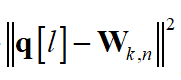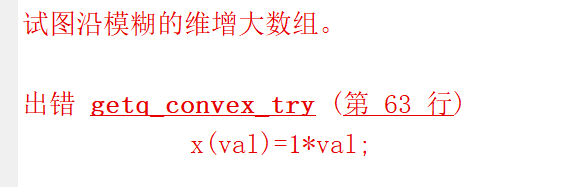# Exhaustive Search Method

Because I couldn’t solve the log problem last time，So I changed the exhaustive search method: when the law of solving the problem is not found, many candidate solutions that may be solutions are enumerated and tested one by one in a certain order, and those candidate solutions that meet the requirements are found as the solution of the problem.

But I can’t think of a proper code to use the method. So I came to get some advice.

(1)Here’s what I think:
I need to find the coordinate point (x,y) of UAV q, which ranges from 0 to 1000m. The user’s coordinate W has been given by randomly scattering points.

This is the calculation I’m going to do:It corresponds to this statement:
re2(1+(i-1)U:iU)=pow_pos((x(val)-w(k,1)),2)+pow_pos((y(val)-w(k,2)),2);

That’s the end of the method: " if abs(M(o)-M(o-1))<delta || o>=Omax break" .

(2-1)Here is my code1:

while 1
cvx_begin
for val=1:10000
x(val)=1val;
y(val)=1
val;
for i=1:2
for k=1:K
re2(1+(i-1)U:iU)=pow_pos((x(val)-w(k,1)),2)+pow_pos((y(val)-w(k,2)),2);
end
end
re=re2’;
val=val+1;
end

cvx_end
[M(o),g(o)]=calcMaxValQ(K,N,t,pSolution,H,re,vSolution,c1,c2,alpha,elta);
if abs(M(o)-M(o-1))<delta || o>=Omax
fprintf(‘The minimum point lie between the interval %d and %d.\n’,x,y);
break
end

But the result: trying to grow the array along the fuzzy dimension.(2-2)Here is my code2:

while 1
cvx_begin

`````` x=0;
y=0;
if x>=0 && x<=1000
if y>=0 && y<=1000

for i=1:2
for k=1:K
re2(1+(i-1)*U:i*U)=pow_pos((x(val)-w(k,1)),2)+pow_pos((y(val)-w(k,2)),2);
end
end
re=re2';

x=x+0.1;
y=y+0.1;
``````

end
end

cvx_end
[M(o),g(o)]=calcMaxValQ(K,N,t,pSolution,H,re,vSolution,c1,c2,alpha,elta);
if abs(M(o)-M(o-1))<delta || o>=Omax
fprintf(‘The minimum point lie between the interval %d and %d.\n’,x,y);
break
end

But the results of my run is x=0.1 and y=0.1. Doesn’t the while loop wait until after the break statement?

This does not appear to actually be CVX code, despite the inclusion of `cvx_begin` and `cvx_end`. There are no CVX variables declared, therefore CVX will do nothing more than declare it an empty model. So your code has nothing to do with CVX; and your exhaustive search method is out of scope of this forum, because it does not involve formulation or solution of any CVX problem.